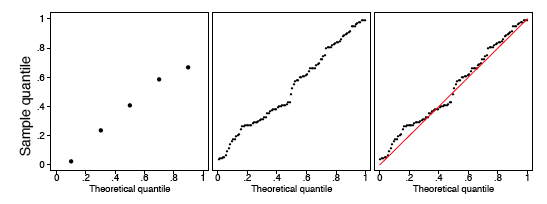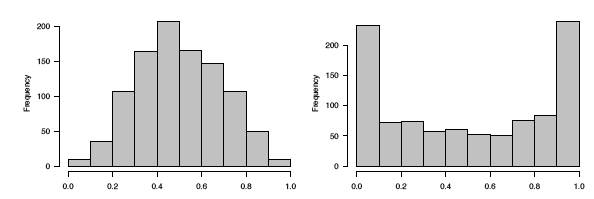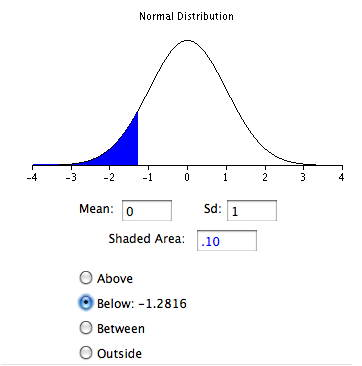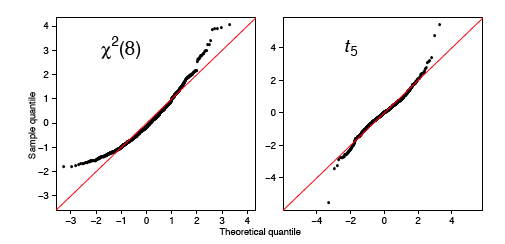# 8.1: Q-Q Plots

•• Contributed by David Lane
• Associate Professor (Psychology, Statistics, and Management) at Rice University

Learning Objectives

• State what $$q-q$$ plots are used for.
• Describe the shape of a $$q-q$$ plot when the distributional assumption is met.
• Be able to create a normal $$q-q$$ plot.

The quantile-quantile or $$q-q$$ plot is an exploratory graphical device used to check the validity of a distributional assumption for a data set. In general, the basic idea is to compute the theoretically expected value for each data point based on the distribution in question. If the data indeed follow the assumed distribution, then the points on the $$q-q$$ plot will fall approximately on a straight line.

Before delving into the details of $$q-q$$ plots, we first describe two related graphical methods for assessing distributional assumptions: the histogram and the cumulative distribution function (CDF). As will be seen, $$q-q$$ plots are more general than these alternatives.

## Assessing Distributional Assumptions

As an example, consider data measured from a physical device such as the spinner depicted in Figure $$\PageIndex{1}$$. The red arrow is spun around the center, and when the arrow stops spinning, the number between $$0$$ and $$1$$ is recorded. Can we determine if the spinner is fair?Figure $$\PageIndex{1}$$: A physical device that gives samples from a uniform distribution

If the spinner is fair, then these numbers should follow a uniform distribution. To investigate whether the spinner is fair, spin the arrow $$n$$ times, and record the measurements by $${\mu _1, \mu _2, ..., \mu _n}$$. In this example, we collect $$n = 100$$ samples. The histogram provides a useful visualization of these data. In Figure $$\PageIndex{2}$$, we display three different histograms on a probability scale. The histogram should be flat for a uniform sample, but the visual perception varies depending on whether the histogram has $$10$$, $$5$$, or $$3$$ bins. The last histogram looks flat, but the other two histograms are not obviously flat. It is not clear which histogram we should base our conclusion on.Figure $$\PageIndex{2}$$:Three histograms of a sample of $$100$$ uniform points

Alternatively, we might use the cumulative distribution function (CDF), which is denoted by $$F(\mu )$$. The CDF gives the probability that the spinner gives a value less than or equal to $$\mu$$, that is, the probability that the red arrow lands in the interval $$[0, \mu ]$$. By simple arithmetic, $$F(\mu ) = \mu$$, which is the diagonal straight line $$y = x$$. The CDF based upon the sample data is called the empirical CDF (ECDF), is denoted by $$\widehat{F}_n(u)$$, and is defined to be the fraction of the data less than or equal to $$\mu$$; that is,

$\widehat{F}_n(u)=\frac{\#u_i\leq u}{n}$

In general, the ECDF takes on a ragged staircase appearance.

For the spinner sample analyzed in Figure $$\PageIndex{2}$$, we computed the ECDF and CDF, which are displayed in Figure $$\PageIndex{3}$$. In the left frame, the ECDF appears close to the line $$y = x$$, shown in the middle frame. In the right frame, we overlay these two curves and verify that they are indeed quite close to each other. Observe that we do not need to specify the number of bins as with the histogram.Figure $$\PageIndex{3}$$: The empirical and theoretical cumulative distribution functions of a sample of $$100$$ uniform points

## q-q plot for uniform data

The $$q-q$$ plot for uniform data is very similar to the empirical CDF graphic, except with the axes reversed. The $$q-q$$ plot provides a visual comparison of the sample quantiles to the corresponding theoretical quantiles. In general, if the points in a $$q-q$$ plot depart from a straight line, then the assumed distribution is called into question.

Here we define the qth quantile of a batch of n numbers as a number $$ξ_q$$ such that a fraction q x n of the sample is less than $$ξ_q$$, while a fraction $$(1 - q) \times n$$ of the sample is greater than $$ξ_q$$. The best known quantile is the median, $$ξ_{0.5}$$, which is located in the middle of the sample.

Consider a small sample of $$5$$ numbers from the spinner:

$\mu _1 = 0.41,\; \mu _2 =0.24,\; \mu _3 =0.59,\; \mu _4 =0.03,\; \mu _5 =0.67$

Based upon our description of the spinner, we expect a uniform distribution to model these data. If the sample data were “perfect,” then on average there would be an observation in the middle of each of the $$5$$ intervals: $$0$$ to $$0.2$$, $$0.2$$ to $$0.4$$, $$0.4$$ to $$0.6$$, and so on. Table $$\PageIndex{1}$$ shows the $$5$$ data points (sorted in ascending order) and the theoretically expected value of each based on the assumption that the distribution is uniform (the middle of the interval).

Table $$\PageIndex{1}$$: Computing the Expected Quantile Values

Data (μ) Rank (i) Middle of the ith Interval
0.03 1 0.1
0.24 2 0.3
0.41 3 0.5
0.59 4 0.7
0.67 5 0.9

The theoretical and empirical CDFs are shown in Figure $$\PageIndex{4}$$ and the $$q-q$$ plot is shown in the left frame of Figure $$\PageIndex{5}$$.Figure $$\PageIndex{4}$$: The theoretical and empirical CDFs of a small sample of $$5$$ uniform points, together with the expected values of the $$5$$ points (red dots in the right frame)

In general, we consider the full set of sample quantiles to be the sorted data values

$μ_{(1)} < μ_{(2)} < μ_{(3)} < \ldots < μ_{(n-1)} < μ_{(n)} ,$

where the parentheses in the subscript indicate the data have been ordered. Roughly speaking, we expect the first ordered value to be in the middle of the interval $$(0, 1/n)$$, the second to be in the middle of the interval $$(1/n, 2/n)$$, and the last to be in the middle of the interval $$(\tfrac{n - 1}{n}, 1)$$. Thus, we take as the theoretical quantile the value

$\xi _q=q\approx \frac{i-0.5}{n}$

where $$q$$ corresponds to the $$i^{th}$$ ordered sample value. We subtract the quantity $$0.5$$ so that we are exactly in the middle of the interval $$(\tfrac{i-1}{n}, \tfrac{i}{n})$$. These ideas are depicted in the right frame of Figure $$\PageIndex{4}$$ for our small sample of size $$n = 5$$.

We are now prepared to define the $$q-q$$ plot precisely. First, we compute the n expected values of the data, which we pair with the n data points sorted in ascending order. For the uniform density, the $$q-q$$ plot is composed of the $$n$$ ordered pairs

$(\tfrac{i-0.5}{n}, u_i),\; for\; i=1,2,\cdots ,n$

This definition is slightly different from the ECDF, which includes the points $$(u_i, \tfrac{i}{n})$$. In the left frame of Figure $$\PageIndex{5}$$, we display the $$q-q$$ plot of the $$5$$ points in Table $$\PageIndex{1}$$. In the right two frames of Figure $$\PageIndex{5}$$, we display the $$q-q$$ plot of the same batch of numbers used in Figure $$\PageIndex{2}$$. In the final frame, we add the diagonal line $$y = x$$ as a point of reference.Figure $$\PageIndex{5}$$: (Left) $$q-q$$ plot of the $$5$$ uniform points. (Right) $$q-q$$ plot of a sample of $$100$$ uniform points

The sample size should be taken into account when judging how close the $$q-q$$ plot is to the straight line. We show two other uniform samples of size $$n = 10$$ and $$n = 1000$$ in Figure $$\PageIndex{6}$$. Observe that the $$q-q$$ plot when $$n = 1000$$ is almost identical to the line $$y = x$$, while such is not the case when the sample size is only $$n = 10$$.Figure $$\PageIndex{6}$$: $$q-q$$ plots of a sample of $$10$$ and $$1000$$ uniform points

In Figure $$\PageIndex{7}$$, we show the $$q-q$$ plots of two random samples that are not uniform. In both examples, the sample quantiles match the theoretical quantiles only at the median and at the extremes. Both samples seem to be symmetric around the median. But the data in the left frame are closer to the median than would be expected if the data were uniform. The data in the right frame are further from the median than would be expected if the data were uniform.Figure $$\PageIndex{7}$$. $$q-q$$ plots of two samples of size $$1000$$ that are not uniform.

In fact, the data were generated in the $$R$$ language from beta distributions with parameters $$a = b = 3$$ on the left and $$a = b =0.4$$ on the right. In Figure $$\PageIndex{8}$$ we display histograms of these two data sets, which serve to clarify the true shapes of the densities. These are clearly non-uniform.Figure $$\PageIndex{8}$$: Histograms of the two non-uniform data sets

## q-q plot for normal data

The definition of the $$q-q$$ plot may be extended to any continuous density. The $$q-q$$ plot will be close to a straight line if the assumed density is correct. Because the cumulative distribution function of the uniform density was a straight line, the $$q-q$$ plot was very easy to construct. For data that are not uniform, the theoretical quantiles must be computed in a different manner.

Let $${z_1, z_2, ..., z_n}$$ denote a random sample from a normal distribution with mean $$\mu =0$$ and standard deviation $$\sigma =1$$. Let the ordered values be denoted by

$z_{(1)} < z_{(2)} < z_{(3)} < \ldots < z_{(n-1)} <z_{(n)}$

These n ordered values will play the role of the sample quantiles.

Let us consider a sample of $$5$$ values from a distribution to see how they compare with what would be expected for a normal distribution. The $$5$$ values in ascending order are shown in the first column of Table $$\PageIndex{2}$$.

Table $$\PageIndex{2}$$: Computing the expected quantile values for normal data.
Data (z) Rank (i) Middle of the ith Interval Normal(z)
-1.96 1 0.1 -1.28
-0.78 2 0.3 -0.52
0.31 3 0.5 0.00
1.15 4 0.7 0.52
1.62 5 0.9 1.28

Just as in the case of the uniform distribution, we have $$5$$ intervals. However, with a normal distribution the theoretical quantile is not the middle of the interval but rather the inverse of the normal distribution for the middle of the interval. Taking the first interval as an example, we want to know the $$z$$ value such that $$0.1$$ of the area in the normal distribution is below $$z$$. This can be computed using the Inverse Normal Calculator as shown in Figure $$\PageIndex{9}$$. Simply set the “Shaded Area” field to the middle of the interval ($$0.1$$) and click on the “Below” button. The result is $$-1.28$$. Therefore, $$10\%$$ of the distribution is below a $$z$$ value of $$-1.28$$.Figure $$\PageIndex{9}$$: Example of the Inverse Normal Calculator for finding a value of the expected quantile from a normal distribution

The $$q-q$$ plot for the data in Table $$\PageIndex{2}$$ is shown in the left frame of Figure $$\PageIndex{11}$$.

In general, what should we take as the corresponding theoretical quantiles? Let the cumulative distribution function of the normal density be denoted by $$\Phi (z)$$. In the previous example, $$\Phi (-1.28)=0.10$$ and $$\Phi (0.00)=0.50$$. Using the quantile notation, if $$\xi _q$$ is the $$q^{th}$$ quantile of a normal distribution, then

$Φ(ξ_q)= q$

That is, the probability a normal sample is less than $$\xi _q$$ is in fact just $$q$$.

Consider the first ordered value, $$z_1$$. What might we expect the value of $$\Phi (z_1)$$ to be? Intuitively, we expect this probability to take on a value in the interval $$(0, 1/n)$$. Likewise, we expect $$\Phi (z_2)$$ to take on a value in the interval $$(1/n, 2/n)$$. Continuing, we expect $$\Phi (z_n)$$ to fall in the interval $$((n - 1)/n, 1)$$. Thus, the theoretical quantile we desire is defined by the inverse (not reciprocal) of the normal CDF. In particular, the theoretical quantile corresponding to the empirical quantile $$z_i$$ should be

$ξ_q \approx \dfrac{i-0.5}{n}$

for $$i = 1, 2, \ldots, n$$.

The empirical CDF and theoretical quantile construction for the small sample given in Table $$\PageIndex{2}$$ are displayed in Figure $$\PageIndex{10}$$. For the larger sample of size $$100$$, the first few expected quantiles are $$-2.576$$, $$-2.170$$, and $$-1.960$$.Figure $$\PageIndex{10}$$: The empirical CDF of a small sample of $$5$$ normal points, together with the expected values of the $$5$$ points (red dots in the right frame)

In the left frame of Figure $$\PageIndex{11}$$, we display the $$q-q$$ plot of the small normal sample given in Table $$\PageIndex{2}$$. The remaining frames in Figure $$\PageIndex{11}$$ display the $$q-q$$ plots of normal random samples of size $$n = 100$$ and $$n = 1000$$. As the sample size increases, the points in the $$q-q$$ plots lie closer to the line $$y = x$$.Figure $$\PageIndex{11}$$: $$q-q$$ plots of normal data

As before, a normal $$q-q$$ plot can indicate departures from normality. The two most common examples are skewed data and data with heavy tails (large kurtosis). In Figure $$\PageIndex{12}$$, we show normal $$q-q$$ plots for a chi-squared (skewed) data set and a Student’s-$$t$$ (kurtotic) data set, both of size $$n = 1000$$. The data were first standardized. The red line is again $$y = x$$. Notice, in particular, that the data from the $$t$$ distribution follow the normal curve fairly closely until the last dozen or so points on each extreme.Figure $$\PageIndex{12}$$: $$q-q$$ plots for standardized non-normal data ($$n = 1000$$)

## q-q plots for normal data with general mean and scale

Our previous discussion of $$q-q$$ plots for normal data all assumed that our data were standardized. One approach to constructing $$q-q$$ plots is to first standardize the data and then proceed as described previously. An alternative is to construct the plot directly from raw data.

In this section, we present a general approach for data that are not standardized. Why did we standardize the data in Figure $$\PageIndex{12}$$? The $$q-q$$ plot is comprised of the $$n$$ points

$\left ( \Phi ^{-1}\left ( \frac{i-5}{n} \right ),z_i \right )\; for\; i=1,2,...,n$

If the original data {$$z_i$$} are normal, but have an arbitrary mean $$\mu$$ and standard deviation $$\sigma$$, then the line $$y = x$$ will not match the expected theoretical quantiles. Clearly, the linear transformation

$μ + σ ξ_q$

would provide the $$q^{th}$$ theoretical quantile on the transformed scale. In practice, with a new data set $$\{x_1,x_2, \ldots, x_n\}$$,

the normal $$q-q$$ plot would consist of the n points

$\left ( \Phi ^{-1}\left ( \frac{i-5}{n} \right ),x_i \right )\; for\; i=1,2,...,n$

Instead of plotting the line $$y = x$$ as a reference line, the line

$y = M + s · x$

should be composed, where $$M$$ and $$s$$ are the sample moments (mean and standard deviation) corresponding to the theoretical moments $$\mu$$ and $$\sigma$$. Alternatively, if the data are standardized, then the line $$y = x$$ would be appropriate, since now the sample mean would be $$0$$ and the sample standard deviation would be $$1$$.

Example $$\PageIndex{1}$$: SAT Case Study

The SAT case study followed the academic achievements of $$105$$ college students majoring in computer science. The first variable is their verbal SAT score and the second is their grade point average (GPA) at the university level. Before we compute inferential statistics using these variables, we should check if their distributions are normal. In Figure $$\PageIndex{13}$$, we display the $$q-q$$ plots of the verbal SAT and university GPA variables.Figure $$\PageIndex{13}$$: $$q-q$$ plots for the student data ($$n = 105$$).

The verbal SAT seems to follow a normal distribution reasonably well, except in the extreme tails. However, the university GPA variable is highly non-normal. Compare the GPA $$q-q$$ plot to the simulation in the right frame of Figure $$\PageIndex{7}$$. These figures are very similar, except for the region where $$x\approx -1$$. To follow these ideas, we computed histograms of the variables and their scatter diagram in Figure $$\PageIndex{14}$$. These figures tell quite a different story. The university GPA is bimodal, with about $$20\%$$ of the students falling into a separate cluster with a grade of $$C$$. The scatter diagram is quite unusual. While the students in this cluster all have below average verbal SAT scores, there are as many students with low SAT scores whose GPAs were quite respectable. We might speculate as to the cause(s): different distractions, different study habits, but it would only be speculation. But observe that the raw correlation between verbal SAT and GPA is a rather high $$0.65$$, but when we exclude the cluster, the correlation for the remaining $$86$$ students falls a little to $$0.59$$.Figure $$\PageIndex{14}$$: Histograms and scatter diagram of the verbal SAT and GPA variables for the $$105$$ students.

## Discussion

Parametric modeling usually involves making assumptions about the shape of data, or the shape of residuals from a regression fit. Verifying such assumptions can take many forms, but an exploration of the shape using histograms and $$q-q$$ plots is very effective. The $$q-q$$ plot does not have any design parameters such as the number of bins for a histogram.

In an advanced treatment, the $$q-q$$ plot can be used to formally test the null hypothesis that the data are normal. This is done by computing the correlation coefficient of the $$n$$ points in the $$q-q$$ plot. Depending upon $$n$$, the null hypothesis is rejected if the correlation coefficient is less than a threshold. The threshold is already quite close to $$0.95$$ for modest sample sizes.

We have seen that the $$q-q$$ plot for uniform data is very closely related to the empirical cumulative distribution function. For general density functions, the so-called probability integral transform takes a random variable $$X$$ and maps it to the interval ($$0, 1$$) through the CDF of $$X$$ itself, that is,

$Y = F_X(X)$

which has been shown to be a uniform density. This explains why the $$q-q$$ plot on standardized data is always close to the line $$y = x$$ when the model is correct.

Finally, scientists have used special graph paper for years to make relationships linear (straight lines). The most common example used to be semi-log paper, on which points following the formula $$y=ae^{bx}$$ appear linear. This follows of course since $$log(y) = log(a) + bx$$, which is the equation for a straight line. The $$q-q$$ plots may be thought of as being “probability graph paper” that makes a plot of the ordered data values into a straight line. Every density has its own special probability graph paper.

## Contributor

• Online Statistics Education: A Multimedia Course of Study (http://onlinestatbook.com/). Project Leader: David M. Lane, Rice University.